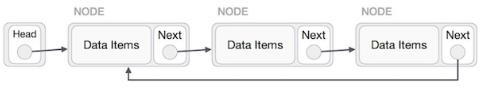# Count nodes in Circular linked list in C++

We are given a circular linked list with the nodes and the task is to calculate the count of nodes present in a circular linked list.

Circular Linked List is a variation of Linked list in which the first element points to the last element and the last element points to the first element. Both Singly Linked List and Doubly Linked List can be made into a circular linked list.

In the below program, we are implementing a singly linked list as a circular linked list to calculate the count of nodes in that.## For Example

Input − nodes-: 20, 1, 2, 3, 4, 5
Output − count of nodes are-: 6
Input − nodes-: 20, 1, 2, 3, 4, 5, 7, 8, 9, 12
Output − count of nodes are-: 10

Approach used in the below program is as follows −

• Create the structure for a singly linked list including the address and data held by the node.

• Create a push() function that will be used to insert the data into the node.

• In the last node, store the address of the first node to make a singly linked list function as a circular linked list.

• Create a count function that will count the total number of nodes present in a circular linked list.

## Example

Live Demo

#include <stdio.h>
#include <stdlib.h>
/* Defining a node */
struct node {
int data;
struct node* next;
};
// Inserting node in Circular list
void push(struct node** head_ref, int data){
struct node* ptr1 = (struct node*)malloc(sizeof(struct node));
ptr1->data = data;
// going to the last node to insert new element.
temp = temp->next;
}
temp->next = ptr1;
} else{
ptr1->next = ptr1; //for first node
}
}
// Function to count the number of nodes
int result = 0;
do {
temp = temp->next;
result++;
}
return result;
}
int main(){
/* Initializing the list as empty */
}
count of nodes are: 4